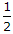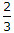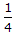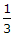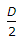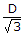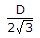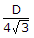# Civil Engineering - Theory of Structures

Exercise : Theory of Structures - Section 4
16.
The degree of indeterminacy of the frame in the given figure, is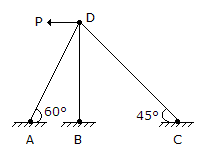zero
1
2
3
Explanation:
No answer description is available. Let's discuss.

17.
The equivalent length of a column of length L having one end fixed and the other end free, is
2L
L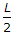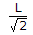Explanation:
No answer description is available. Let's discuss.

18.
To determine the force in BD of the truss shown in the given figure a section is passed through BD, CD and CE, and the moments are taken about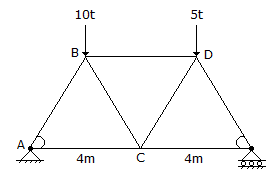A joint
B joint
C joint
D joint.
Explanation:
No answer description is available. Let's discuss.

19.
The ratio of the length and depth of a simply supported rectangular beam which experiences maximum bending stress equal to tensile stress, due to same load at its mid span, is